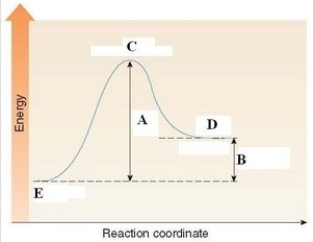Energy Changes,reaction Rates,and Equilibrium

# General Organic and Biological Chemistry Study Set 1

Chemistry

## Quiz 6 :Energy Changes,reaction Rates,and EquilibriumStudy FlashcardsLooking for Organic Chemistry Homework Help?## Quiz 6 :Energy Changes,reaction Rates,and Equilibrium

Showing 1 - 20 of 102The law of conservation of energy states that ________.
Free
Multiple Choice

DA chemical reaction requires 31.39 kJ. How many kilocalories does this correspond to?
Free
Multiple Choice

BA chemical reaction releases 55.2 kcal. How many kilojoules does this correspond to?
Free
Multiple Choice

AWhich of the following energy quantities is equivalent to 578 J?
Multiple ChoiceWhich of the following energy quantities is equivalent to 11.9 kcal?
Multiple ChoiceWhich of the following energy quantities is equivalent to 258 Cal?
Multiple ChoiceWhich quantity represents the largest amount of stored energy?
Multiple ChoiceA peanut butter and jelly sandwich contains 9.00 g of fat,6.00 g of protein,and 25.0 g of carbohydrate. How many Calories does this sandwich provide?
Multiple ChoiceWhich bond is the strongest?
Multiple ChoiceWhich bond is the weakest?
Multiple ChoiceWhich term correctly describes a reaction in which the energy of the products is higher than the energy of the reactants?
Multiple ChoiceConsider the reaction: C3H8(g)+ 5 O2(g)→ 3 CO2(g)+ 4 H2O(g),where ΔH = -531 kcal. Which statement concerning this reaction is true?
Multiple ChoiceConsider the reaction,C2H4(g)+ H2(g)→ C2H6(g),where ΔH = -137 kJ. How many kilojoules are released when 3.5 mol of C2H4 reacts?
Multiple ChoiceConsider the reaction,C2H4(g)+ H2(g)→ C2H6(g),where ΔH = -137 kJ. How many kilojoules are released when 55.3 g of C2H4 reacts?
Multiple ChoiceConsider the reaction,3 NO2(g)+ H2O(l)→ 2 HNO3(aq)+ NO(g),where ΔH = -137 kJ. How many kilojoules are released when 92.3 g of NO2 reacts?
Multiple ChoiceWhich of the following is a true statement about the energy diagram shown below?Multiple ChoiceIn the reaction: 2 C2H6(g)+ 7 O2(g)→ 4 CO2(g)+ 6 H2O(g),increasing the concentration of C2H6(g)will ________.
Multiple ChoiceCatalysts accelerate a reaction by ________.
Multiple Choice